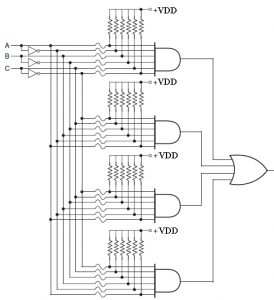Home > programmable logic devices

# Programmable Logic Devices

Two categories of digital logic devices are fixed logic devices and programmable logic devices. In fixed logic devices user can implement the fixed combinational or sequential circuit. In the other hand, in programmable logic device user can implement various functions.
Programmable logic device implements wide range of logic functions. Figure below shows a fixed logic circuit of AND and OR gate. This circuit produces an output that is the sum output of a full adder.Figure below shows the logic diagram of a programmable logic device. The device has an array of AND gates at the input and a OR gate at the output. Here, the AND gate handles three variables which produces product term of three variables. The A, B and C inputs of AND gates are fusible links called antifuses. Hence, each AND gate produce the product term of A, B and C inputs.